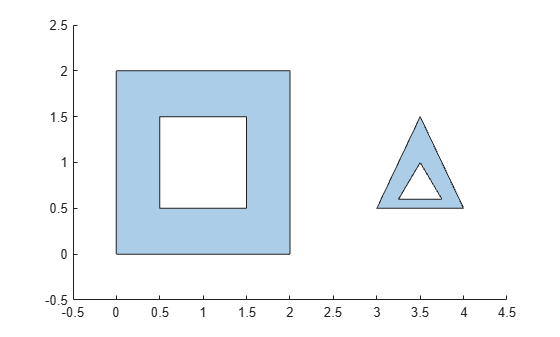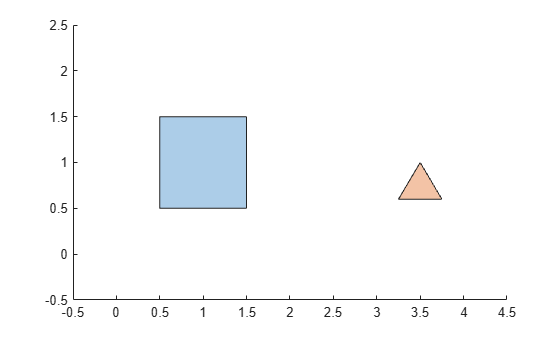# holes

Convert `polyshape` hole boundaries to array of `polyshape` objects

## Syntax

``polyout = holes(polyin)``

## Description

example

````polyout = holes(polyin)` returns the hole boundaries of a `polyshape` object as an array of `polyshape` objects. The number of elements in `polyout` is equal to the number of hole boundaries in `polyin`.```

## Examples

collapse all

Create a polygon containing two solid regions and two holes. Convert the hole boundaries to an array of `polyshape` objects.

```P = [0 0; 0 2; 2 2; 2 0; NaN NaN; 0.5 0.5; 0.5 1.5; 1.5 1.5; 1.5 0.5; ... NaN NaN; 3 0.5; 3.5 1.5; 4 0.5; NaN NaN; 3.25 0.6; 3.75 0.6; 3.5 1]; polyin = polyshape(P); plot(polyin) xlim([-0.5 4.5]) ylim([-0.5 2.5])````polyout = holes(polyin)`
```polyout = 2x1 polyshape array with properties: Vertices NumRegions NumHoles ```
```plot(polyout) xlim([-0.5 4.5]) ylim([-0.5 2.5])```## Input Arguments

collapse all

Input `polyshape`, specified as a scalar.

Data Types: `polyshape`